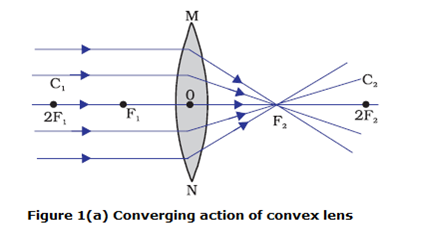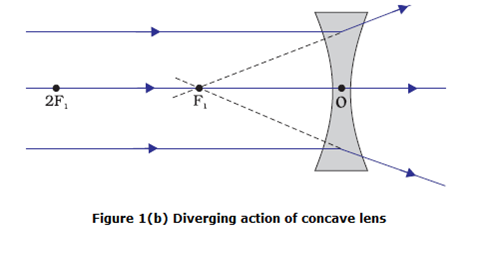# Refraction by spherical lens and image formation by lens

## Refraction by Spherical Lenses

• A Spherical lens is a piece of transparent glass bound by two spherical surfaces.
• There are two types of Spherical Lenses
• A convex lens bulges outward and is thick at the center and thinner at the edges. Convex lens converges the light rays as shown below in the figure 1(a).Hence convex lenses are called converging lenses.
• A concave lens bulges inward and is thinner in the middle and thicker at the edges. Such lenses diverge light rays as shown in Figure 1(b)Such lenses are called diverging lenses.
• A lens, whether it is a convex lens or a concave lens, has two spherical surfaces which form a part of a sphere. The centers of these spheres are called centers of curvature of the lens usually represented by the letter C.
• Since there are two centers of curvature, we may represent them as C1 and C2.
• An imaginary straight line passing through the two centers of curvature of a lens is called its principal axis as shown in figure 1.
• The central point of a lens is its optical centre. It is usually represented by the letter O.
• A ray of light through the optical centre of a lens passes without suffering any deviation.
• The effective diameter of the circular outline of a spherical lens is called its aperture.
• In figure 1 (a) you can see several rays of light parallel to the principal axis are falling on a convex lens. These rays, after refraction from the lens, are converging to a point on the principal axis. This point on the principal axis is called the principal focus of the lens.
• Letter F is usually used to represent principal focus. A lens has two principal foci.
• Similarly in figure 1 (b) several rays of light parallel to the principal axis are falling on a concave lens. These rays, after refraction from the lens, are appearing to diverge from a point on the principal axis. This point on the principal axis is called the principal focus of the concave lens.
• The distance of the principal focus from the optical centre of a lens is called its focal length represented by letter f .

## Image Formation by Lenses

• Lenses form images by refraction of light and type of image formation depends on the position of the object in front of the lens.
• We can place the objects at
1. Infinity
2. Beyond 2F1
3. At 2F1
4. Between F1 and 2F1
5. At focus F1
6. Between focus F1 and optical center O
• Image formation by a convex lens for different positions of the object is shown below in the table
Position of the object Position of the image Relative size of the image Nature of the image
Infinity At focus F2 Highly diminished, point sized Real and inverted
Beyond 2F1 Between F2 and 2F2 Diminished Real and inverted
At 2F1 At 2F2 Same size Real and inverted
Between F1 and 2F1 Beyond 2F2 Enlarged Real and inverted
At focus 2F1 At infinity Infinitely large or highly enlarged Real and inverted
Between F1 and optical center O On the same side of the lens as the object Enlarged Virtual and erect
• Nature, position and relative size of the image formed by a concave lens for various positions of the object is given below in the table

• Position of the object Position of the image Relative size of the image Nature of the image
At infinity At focus F Highly diminished, point-sized Virtual and erect
Between infinity and optical center O of the lens Between F1 and optical center O Diminished Virtual and erect
• A concave lens will always give a virtual, erect and diminished image, irrespective of the position of the object.

Given below are the links of some of the reference books for class 10 Science.

You can use above books for extra knowledge and practicing different questions.

### Practice Question

Question 1 Which among the following is not a base?
A) NaOH
B) $NH_4OH$
C) $C_2H_5OH$
D) KOH
Question 2 What is the minimum resistance which can be made using five resistors each of 1/2 Ohm?
A) 1/10 Ohm
B) 1/25 ohm
C) 10 ohm
D) 2 ohm
Question 3 Which of the following statement is incorrect? ?
A) For every hormone there is a gene
B) For production of every enzyme there is a gene
C) For every molecule of fat there is a gene
D) For every protein there is a gene

Note to our visitors :-

Thanks for visiting our website.